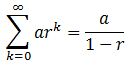# Understand the Formula for Infinite Geometric Series

An error occurred trying to load this video.

Try refreshing the page, or contact customer support.

Coming up next: Using Recursive Rules for Arithmetic, Algebraic & Geometric Sequences

### You're on a roll. Keep up the good work!

Replay
Your next lesson will play in 10 seconds
• 0:01 Infinite Geometric Series
• 1:39 The Formula
• 2:29 Using the Formula
• 3:12 One More Example
• 3:51 Lesson Summary

Want to watch this again later?

Timeline
Autoplay
Autoplay
Speed

#### Recommended Lessons and Courses for You

Lesson Transcript
Instructor: Yuanxin (Amy) Yang Alcocer

Amy has a master's degree in secondary education and has taught math at a public charter high school.

Watch this video lesson to learn how to calculate the sum of an infinite geometric series. Learn about the interesting thing that happens when your common ratio is less than one.

## Infinite Geometric Series

A geometric series is a sequence of numbers where each number is the previous multiplied by a constant, the common ratio. These geometric series go on forever, but most times we are only interested in finding the sum of the beginning part of the series.

For example, say you wanted to spread the word about this huge pool party that you are having at your dream house by the ocean. You send your party invite to five of your friends. Then you ask these five friends to each send the invite to five more people.

Now 25 new people will have an invitation. If these 25 people send the invite to five more people each, your invite will have reached 125 new people. You can see that you only need to add up the first few numbers to get to a really large number for your pool party.

Sometimes, though, you want to see what kind of numbers you get when you add up the infinite series. For example, say that you have a pie and you slice your pie in half. You take one of those slices and slice it in half. You take one of these slices and slice it in half. You keep repeating. Imagine doing this an infinite number of times.

Now you want to add up your pie slices to see how much pie you have. We call this 'when n goes to infinity' since n tells us the number of terms we are talking about. So, if n is infinity, then we are talking about all the terms in our infinite series. In our case, it is all the slices that we have.

It is the sum that we will be talking about in this video lesson. I will show you a formula you can use when your common ratio is within a certain range. So, let's get going!

## The Formula

If your common ratio is less than 1 or greater than -1, but not 0, then you can use this formula to calculate the sum for your infinite geometric series:The r is our common ratio, and the a is the beginning number of our geometric series. To use this formula, our r has to be between -1 and 1, but it cannot be 0. Nor can it be -1 or 1.

So, if our r is 1/2, 1/4, 1/3, etc., or even -1/2, -1/4, -1/3, then we can use this formula. If our r is outside these limits, if it is greater than or equal to 1 or less than or equal to -1, then the sum of the infinite geometric series cannot be evaluated.

To unlock this lesson you must be a Study.com Member.

### Register to view this lesson

Are you a student or a teacher?

### Unlock Your Education

#### See for yourself why 30 million people use Study.com

##### Become a Study.com member and start learning now.
Back
What teachers are saying about Study.com

### Earning College Credit

Did you know… We have over 160 college courses that prepare you to earn credit by exam that is accepted by over 1,500 colleges and universities. You can test out of the first two years of college and save thousands off your degree. Anyone can earn credit-by-exam regardless of age or education level.